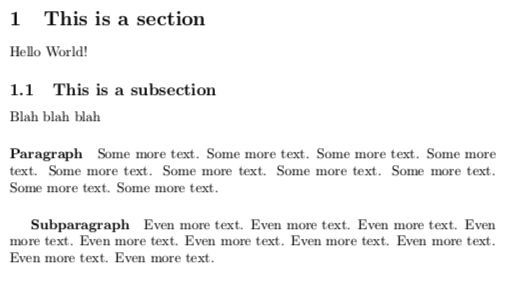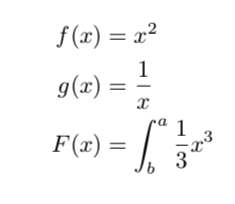{{ message }}

Instantly share code, notes, and snippets.

# tombusby/LaTeX-study-notes.md

Last active Jan 11, 2020
LaTeX Study Notes

# LaTeX Study Notes

## Commands

Commands begin with a \ and are instructions to LaTeX (i.e. not content which will appear on the page - although they can cause content to be generated such as the \LaTeX{} macro).

For example:

\documentclass{article}

## Document Classes

These influence the overall layout of your document. There are many; including article and book.

## Environments

An environment is simply an area of your document where certain typesetting rules apply. It is possible (and usually necessary) to have multiple environments in a document, but it is imperative the document environment is the topmost environment.

\begin{document}
\begin{environment1}
\begin{environment2}
\end{environment2}
\end{environment1}
\end{document}

## Title Pages

These can be generated via the \maketitle macro:

\documentclass{article}

\title{My first document}
\date{2020-01-11}
\author{Thomas Busby}

\begin{document}
\maketitle
\newpage
Hello World!
\end{document}

## Page Numbering

This can be set to gobble (no page numbering), arabic or roman:

\documentclass{article}

\title{My first document}
\date{2020-01-11}
\author{Thomas Busby}

\begin{document}
\pagenumbering{gobble}
\maketitle
\newpage
\pagenumbering{arabic}

Hello World!
\end{document}

## Preamble

A LaTeX document has a preamble part and a document. The preamble in the previous example includes the documentclass, title, author and date.

## Sections, Subsections, Paragraphs and Subparagraphs

Sections allow us to break up our text into automatically-numbered units.

\documentclass{article}

\title{My first document}
\date{2020-01-11}
\author{Thomas Busby}

\begin{document}
\pagenumbering{gobble}
\maketitle
\newpage
\pagenumbering{arabic}

\section{This is a section}

Hello World!

\subsection{This is a subsection}

Blah blah blah

\paragraph{Paragraph}

Some more text. Some more text. Some more text. Some more text. Some more text. Some more text. Some more text. Some more text. Some more text. Some more text.

\subparagraph{Subparagraph}

Even more text. Even more text. Even more text. Even more text. Even more text. Even more text. Even more text. Even more text. Even more text. Even more text.

\end{document}

This produces the following:## Packages

LaTeX is extensible. Packages can be used to import new functionality not provided by default.

To use a package you add a declaration to the preamble:

\documentclass{article}

\usepackage{PACKAGENAME}

\begin{document}
...
\end{document}

## Mathematical Notation

amsmath is a popular package for typesetting equations.

Mathematical equations can be formatted using \begin{equation} (or \begin{equation*} from amsmath when you don't want to give them numbers.

\begin{equation}
f(x) = x^2
\end{equation}

\begin{equation*}
f(x) = x^2
\end{equation*}

When you want to format an equation inline, you wrap it in $ as follows: This formula$f(x) = x^2\$ is an example of inline formulas.

You can use & to force the formatted equations to keep the equals signs in line:

\begin{align*}
f(x) &= x^2\\
g(x) &= \frac{1}{x}\\
F(x) &= \int^a_b \frac{1}{3}x^3
\end{align*}

This produces: